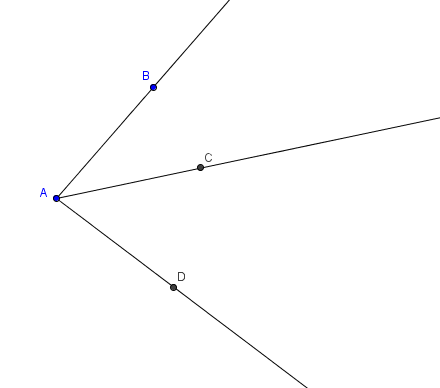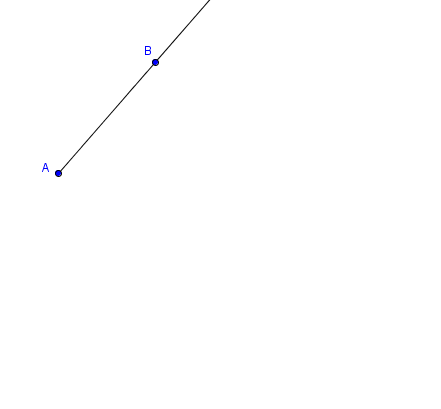﻿ Protractor Worksheet
PROTRACTOR WORKSHEET

First, study these web animations:
Using a Protractor to Measure Angles at: http://www.mathopenref.com/constmeasureangle.html
Animation: Using a Protractor to Draw an Angle at: http://www.mathopenref.com/constdrawangle.htmlUse a protractor to measure the specified angles in the diagram above: the measure of $\,\angle BAC\,$ is __________ the measure of $\,\angle CAD\,$ is __________ the measure of $\,\angle BAD\,$ is __________ Two of your angle measures should sum to the third. Check that this is true:

 Using the diagram below: Use your protractor to draw a $\,37^{\circ}\,$ angle with vertex $\,A\,$ and with $\,\overrightarrow{AB}\,$ as one of its sides. Label a point $\,C\,$ on the other side of the angle you just drew. Use your protractor to draw a $\,49^{\circ}\,$ angle with vertex $\,A\,$ and with $\,\overrightarrow{AC}\,$ as one of its sides.